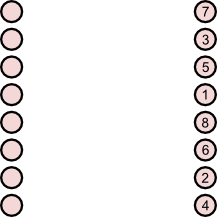### How to button as badly as possible

The following problem was suggested by Bernard Murphy at Jenny Piggott’s retirement meeting in Cambridge on Tuesday 13 July.

### Problem

Consider eight jacket buttons in a column, as shown on the left hand side of the image below.We can unbutton the eight buttons in various orders; one such order is shown on the right hand side of the above figure. The problem is to choose an ordering so that we have to move up and down the buttons the maximum number of times. For example, if we unbutton in the usual fashion, starting from the top button and finishing with the bottom button, we would make in total seven moves (from first button to second button, from second button to third button, and so forth). In the example above, we travel from button 4 to button 7 (3 moves), then button 7 to button 2 (5 moves), and so forth, totalling 31 moves.

Bernard’s question was What is the maximum number of moves? We add to that question Which unbuttoning strategies yield this maximum?

### Solution

I’ll state the answers here, and prove them below. The maximum number of moves is 31. One unbuttoning strategy which yields this solution is shown above. What do the others look like? They can all be described as follows. The four odd labels 1, 3, 5, and 7 must all occur in either the top four, or bottom four, positions. Also, labels 1 and 8 must occupy buttons 4 and 5, in either order.

### Reformulate the problem

A suggestion made at the time was to regard this as a graph theory problem. Let the buttons represent the vertices of a weighted complete graph, and the weight between two buttons is the number of moves it takes to get from one button to the other. The original question then becomes a special case of a travelling salesman problem. I see this perspective as a significant backwards step; we have moved from a simple linear graph to a complicated complete graph, and I won’t discuss this view any further.

A more obvious graph theoretic model is to consider the buttons to be vertices of the linear graph shown below. If we equip this graph with the usual graph metric d, then the problem is to choose an ordering v1,…,v8 of the vertices such that the quantity d(v1,v2)+d(v2,v3)+…+d(v7,v8) is maximised.A third form of the problem is to choose an ordering x1,…,x8 of the integers 1,…,8 such that the sum |x1-x2|+|x2-x3|+…+|x7-x8| is maximised. (Here xi is the number of the ith button chosen.) I solve this problem in the next section.

### Explanation of solution

We first solve a more symmetric version of the last problem, which is to choose an ordering x1,…,x8 of the integers 1,…,8 such that the sum |x1-x2|+|x2-x3|+…+|x7-x8|+|x8-x1| is maximised. There is an extra final term in the sum, which corresponds to moving from the finish button back to the start button.

Let S=|x1-x2|+|x2-x3|+…+|x7-x8|+|x8-x1|. The numbers 1,…,8 each occur twice as terms xi in this sum. Consider, for each i, reordering the terms xi and xi+1 from each summand |xi-xi+1|, if necessary, so that the modulus sign can be removed without affecting the value of S. We end up with eight +xi terms, and eight -xi terms. The maximum value of S is attained if and only if the eight plus terms are 5, 6, 7, and 8 (each twice) and the eight minus terms are 1, 2, 3, and 4 (each twice). Thus this maximum is attained if and only if we repeatedly alternate between the top four and bottom four buttons. The maximum has value 2×((5+6+7+8)-(1+2+3+4))=32.

Our simpler, but less symmetric, problem cannot, therefore, have value greater than 31. Since we know that 31 can be achieved, it is the maximum value for the problem. To obtain all the orderings which yield this value 31 we simply select those solutions which have |x8-x1|=1. Now, |x8-x1|=1 if and only if x1 and x8, the start and finish buttons, occupy the middle two button positions.